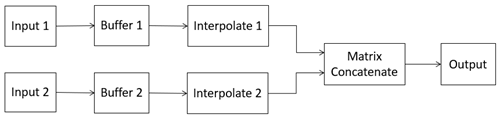# Sample-Rate Match

Upsample two signals to common rate

• Library:
• Communications Toolbox / RF Impairments and Components

•## Description

The Sample-Rate Match block upsamples the input signals, as needed, to a common output sample rate. This block introduces delays to your simulation. Use the `srmdelay` function to compute the delay that will be introduced.

## Ports

### Input

expand all

First input signal, specified as a column vector or a K1-by-Nchan1 matrix. K1 is the number of rows and Nchan1 is the number of channels in the input signal matrix.

Data Types: `single` | `double`
Complex Number Support: Yes

Second input signal, specified as a column vector or a K2-by-Nchan2 matrix. K2 is the number of rows and Nchan2 is the number of channels in the input signal matrix.

Data Types: `single` | `double`
Complex Number Support: Yes

### Output

expand all

Output signal, returned as a P-by-(Nchan1+Nchan2) matrix of the same data type as the input signals. P is the ```Output samples per frame``` parameter value.

Data Types: `single` | `double`
Complex Number Support: Yes

## Parameters

expand all

Sample rate of each input signal in Hz, specified as a 2-element vector of positive values.

Number of samples output per frame, specified as a positive integer.

Options for the output sample rate, specified as either `Auto` or `Specify via property`.

Output signal sample rate in Hz, specified as a positive scalar. The value must be greater than or equal to the ```Input sample rate (Hz)``` parameter values.

#### Dependencies

To enable this parameter, set the ```Output sample rate options``` parameter to `Specify via property`.

## Block Characteristics

 Data Types `double` | `single` Multidimensional Signals `no` Variable-Size Signals `no`

## Algorithms

This figure illustrates the algorithm processing of input signal data for the sample rate match.The input signals are first buffered to a common length specified by the ```Output samples per frame``` parameter. The buffered signals are interpolated to match their sample rates to the output sample rate. The two interpolated signals are then concatenated to produce the matrix output. The interpolation and decimation factors of the interpolation operation are computed as [L,M] = `rat`(RO/RI, 0), where:

• RO is the output sample rate, which is either automatically computed or specified in Output sample rate (Hz).

• RI is the input sample rate specified in Input sample rate (Hz).

• L is the interpolation factor.

• M is the decimation factor.

## Version History

Introduced in R2022b